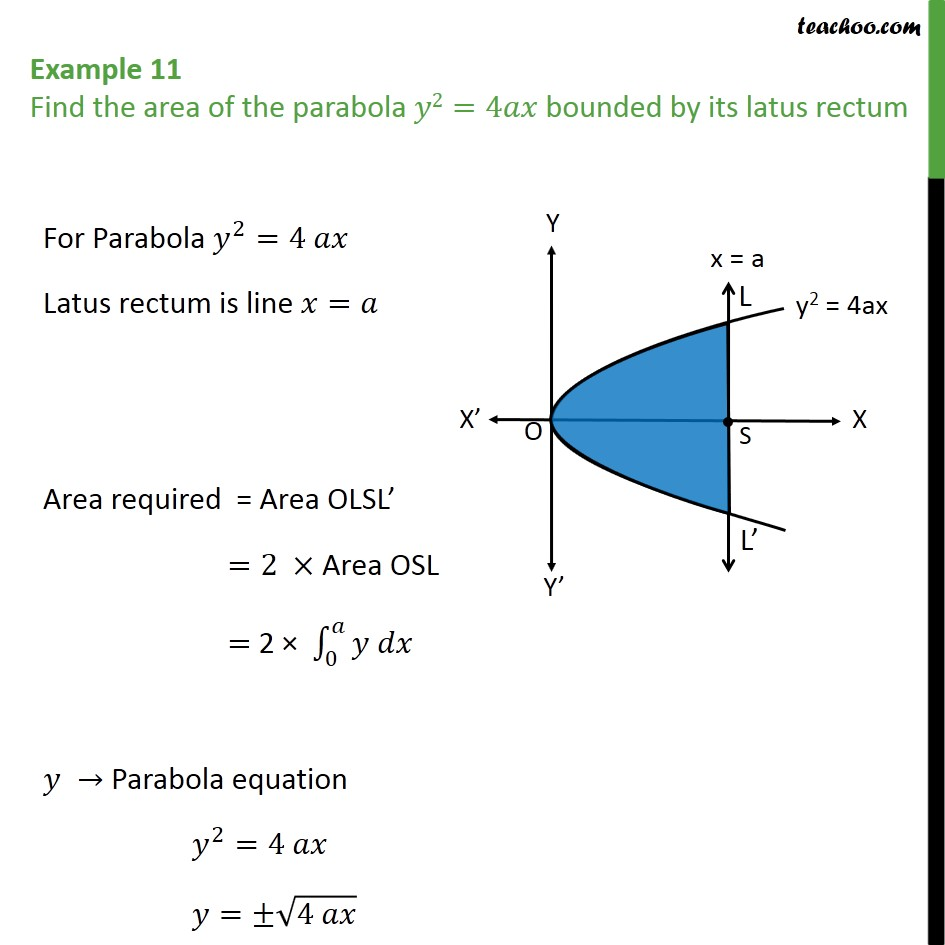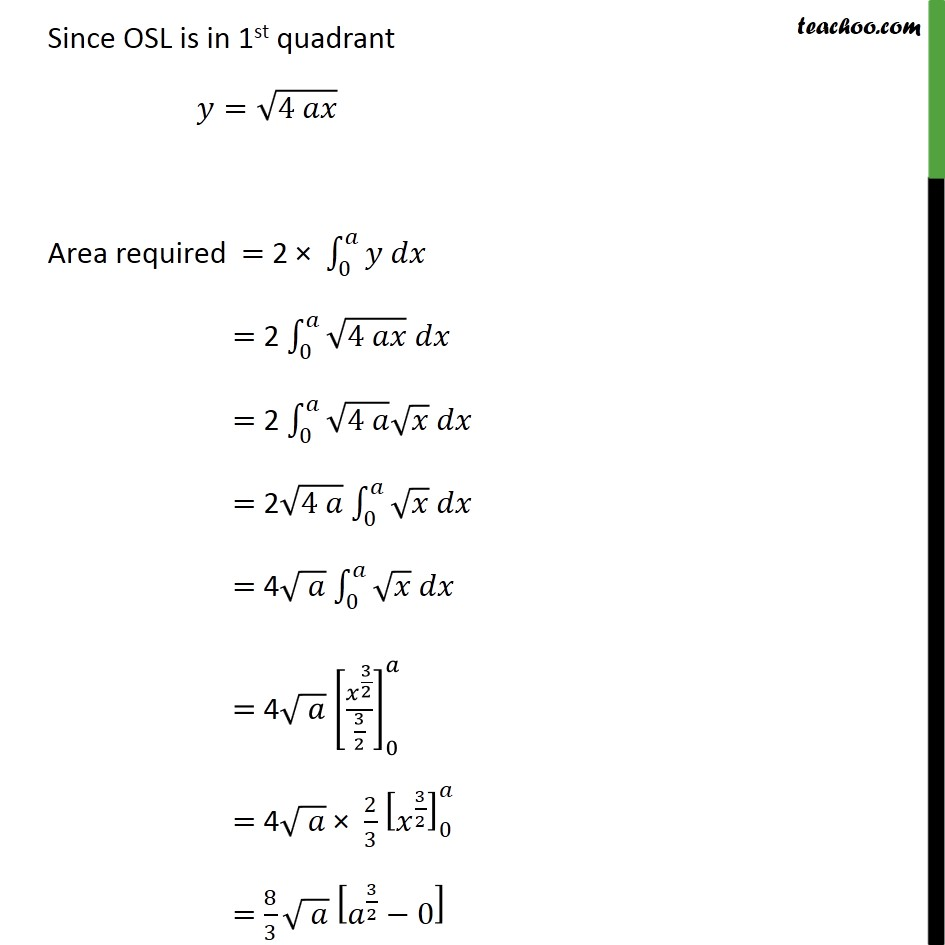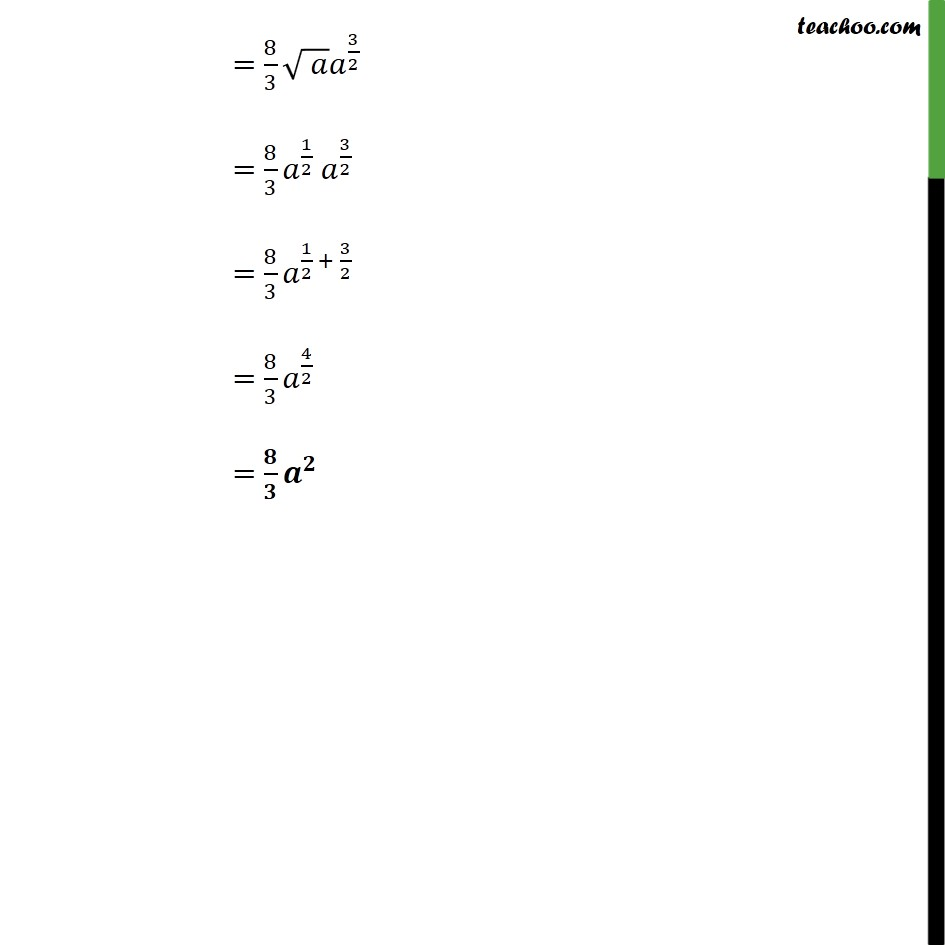Area bounded by curve and horizontal or vertical line

Chapter 8 Class 12 Application of Integrals
Concept wiseLearn in your speed, with individual attention - Teachoo Maths 1-on-1 Class

### Transcript

Question 9 Find the area of the parabola 2=4 bounded by its latus rectum For Parabola 2 =4 Latus rectum is line = Area required = Area OLSL =2 Area OSL = 2 0 Parabola equation 2 =4 = 4 Since OSL is in 1st quadrant = 4 Area required = 2 0 = 2 0 4 = 2 0 4 = 2 4 0 = 4 0 = 4 3 2 3 2 0 = 4 2 3 3 2 0 = 8 3 3 2 0 = 8 3 3 2 = 8 3 1 2 3 2 = 8 3 1 2 + 3 2 = 8 3 4 2 =